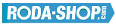# Remote ControlManufacturer Product Image Item Name Price### 623-2Z

Miniature Metric Series Chrome Steel Metallic Shields on both sides Dimension d=3.00 Dimension D=10.00 Dimension B=4.00 Dinamic Load Rating=631 Static Load Rating=219 Weight=0.002gr. Speed limit Grease=50.0 Speed limit Oil=60.0
inside diameter = 3.00
outside diameter = 10.00
width = 4.00
€2.24### 624

Miniature Metric Series Chrome Steel Open(No Shields) Dimension d=4.00 Dimension D=13.00 Dimension B=5.00 Dinamic Load Rating=1301 Static Load Rating=488 Weight=0.004gr. Speed limit Grease=40.0 Speed limit Oil=48.0
inside diameter = 4.00
outside diameter = 13.00
width = 5.00
€2.24### 624-2RS

Miniature Metric Series Chrome Steel Rubber Seals on Both sides Dimension d=4.00 Dimension D=13.00 Dimension B=5.00 Dinamic Load Rating=1301 Static Load Rating=488 Weight=0.004gr. Speed limit Grease=40.0 Speed limit Oil=48.0
inside diameter = 4.00
outside diameter = 13.00
width = 5.00
€2.24### 624-2Z

Miniature Metric Series Chrome Steel Metallic Shields on both sides Dimension d=4.00 Dimension D=13.00 Dimension B=5.00 Dinamic Load Rating=1301 Static Load Rating=488 Weight=0.004gr. Speed limit Grease=40.0 Speed limit Oil=48.0
inside diameter = 4.00
outside diameter = 13.00
width = 5.00
€2.24### 625

Miniature Metric Series Chrome Steel Open(No Shields) Dimension d=5.00 Dimension D=16.00 Dimension B=5.00 Dinamic Load Rating=1729 Static Load Rating=675 Weight=0.006gr. Speed limit Grease=36.0 Speed limit Oil=43.0
inside diameter = 5.00
outside diameter = 16.00
width = 5.00
€2.24### 625-2RS

Miniature Metric Series Chrome Steel Rubber Seals on Both sides Dimension d=5.00 Dimension D=16.00 Dimension B=5.00 Dinamic Load Rating=1729 Static Load Rating=675 Weight=0.006gr. Speed limit Grease=36.0 Speed limit Oil=43.0
inside diameter = 5.00
outside diameter = 16.00
width = 5.00
€2.24### 625-2Z

Miniature Metric Series Chrome Steel Metallic Shields on both sides Dimension d=5.00 Dimension D=16.00 Dimension B=5.00 Dinamic Load Rating=1729 Static Load Rating=675 Weight=0.006gr. Speed limit Grease=36.0 Speed limit Oil=43.0
inside diameter = 5.00
outside diameter = 16.00
width = 5.00
€2.24### 626

Miniature Metric Series Chrome Steel Open(No Shields) Dimension d=6.00 Dimension D=19.00 Dimension B=6.00 Dinamic Load Rating=2336 Static Load Rating=896 Weight=0.008gr. Speed limit Grease=32.0 Speed limit Oil=40.0
inside diameter = 6.00
outside diameter = 19.00
width = 6.00
€2.24### 626-2RS

Miniature Metric Series Chrome Steel Rubber Seals on Both sides Dimension d=6.00 Dimension D=19.00 Dimension B=6.00 Dinamic Load Rating=2336 Static Load Rating=896 Weight=0.008gr. Speed limit Grease=32.0 Speed limit Oil=40.0
inside diameter = 6.00
outside diameter = 19.00
width = 6.00
€2.24### 626-2Z

Miniature Metric Series Chrome Steel Metallic Shields on both sides Dimension d=6.00 Dimension D=19.00 Dimension B=6.00 Dinamic Load Rating=2336 Static Load Rating=896 Weight=0.008gr. Speed limit Grease=32.0 Speed limit Oil=40.0
inside diameter = 6.00
outside diameter = 19.00
width = 6.00
€2.24